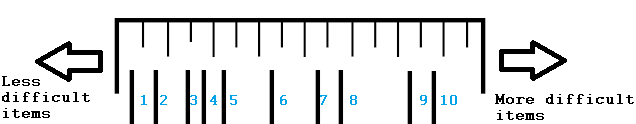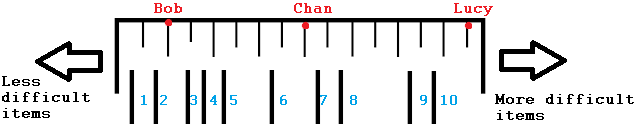# Rasch Model / Rasch Analysis: Definition, Examples

Item Response Theory > Rasch Analysis

## What is a Rasch Model?

The Rasch model is used to measure latent traits like attitude or ability; It shows the probability of an individual getting a correct response on a test item.

The Rasch model is a lot simpler and intuitive than many other models. It resembles a ruler, only instead of cm or inches, the scale is measured with “items.” Less difficult items are to the left, more difficult items are to the right.People can also be placed on the same measurement scale, with less able people on the left and more able people on the right. Let’s add three people to the scale: Bob (a poor candidate), Chan (fairly good) and Lucy (excellent):From the scale, you should be able to tell that Bob is expected to get 2/10 questions right (items 1 and 2), Chan is expected to get 6/10 right and Lucy 10/10. For the Rasch model to work correctly, the tests must be un-timed, otherwise you’ll be adding a further factor into the mix (the probability of attempting the item).

The model is created from actual data — the proportion of responses of each person to each test item. Therefore, the scale is only dependent on the test item’s difficulty and the person’s ability.

## Rasch vs. IRT

Unlike Item Response Theory (IRT), which fits a model to data, a Rasch model is created from the data itself. In addition, general IRT theory uses three parameters, while the Rasch model only uses one. Due to these differences, some schools of thought do not think Rasch and IRT belong in the same category — although you’ll also see plenty of people who do. In essence, it doesn’t matter much if they are in the same category or not, as long as you are applying the theory correctly.

References:
Bond TG, Fox CM. Applying the Rasch model: fundamental measurement in the human sciences. Mahwah: Lawrence Erlbaum Associates; 2001.
Slinde, J, Linn, R. The Rasch Model, Objective Measurement,Equating, and Robustness. Available here.

CITE THIS AS:
Stephanie Glen. "Rasch Model / Rasch Analysis: Definition, Examples" From StatisticsHowTo.com: Elementary Statistics for the rest of us! https://www.statisticshowto.com/rasch-model/